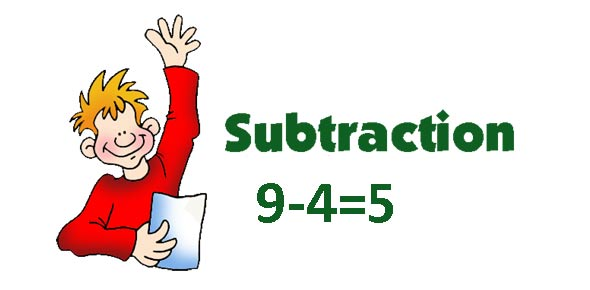25 Questions | Total Attempts: 32Settings.

Related Topics
• 1.
Name:
• 2.
• 3.
• 4.
• 5.
• 6.
• 7.
• 8.
• 9.
• 10.
• 11.
Write the answer for each subtraction sum below:8-1 = _______
• 12.
Write the answer for each subtraction sum below:9-7 = _______
• 13.
Write the answer for each subtraction sum below:10-6 = _______
• 14.
Write the answer for each subtraction sum below:11-7 = _______
• 15.
Calculate the following sum:  55+25
• 16.
Calculate the following sum:  45+36
• 17.
Calculate the following sum:  38+23
• 18.
Calculate the following sum:  652+253
• 19.
Calculate the following sum:  345+357
• 20.
Calculate the following sum:  289+203
• 21.
Using the table below, create sums to find out each answer: RubyGirl12MikalaGirl14SarahGirl11BrainBoy10BrentBoy9AndrewBoy9How many books did they read altogether?
• 22.
How many books did the boys read?
• 23.
What is the difference between the number of books the boys read and the number the girls read?
• 24.
Calculate the following sum:  756    43+  70
• 25.
Calculate the following sum:      2  289+467